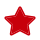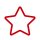# matmul

#### 功能说明

tensor_a与tensor_b的shape后两维(经过对应转置)需要满足矩阵乘(M, K) * (K, N) = (M, N)，支持多维度。如果is_fractal置为True的话，tensor_a数据排布要满足L0A的分形结构，tensor_b要满足L0B的分形结构。如果is_fractal置为False，tensor_a, tensor_b都是ND排布结构。

#### 函数原型

te.lang.cce.matmul(tensor_a, tensor_b, trans_a=False, trans_b=False, format_a="ND", format_b="ND", alpha_num=1.0, beta_num=0.0, dst_dtype="float16", tensor_bias=None, quantize_params=None)

#### 参数说明

• tensor_a：A矩阵，tvm.tensor类型。
• tensor_b：B矩阵，tvm.tensor类型
• trans_a：A矩阵是否转置，bool类型。
• trans_b：B矩阵是否转置，bool类型。
• format_a：输入矩阵A的数据排布格式，取值为：“ND”“fractal”
• format_b：输入矩阵B的数据排布格式，取值为：“ND”“fractal”
• alpha_num：扩展参数，当前暂不使用，默认值1.0。
• beta_num：扩展参数，当前暂不使用，默认值0.0。
• dst_dtype：输出数据类型，支持：float16与float32。
• quantize_params：量化相关参数的。
量化相关入参，采用字典格式。quantize_params为None表示去使能量化，非None表示使能量化，包含以下参数：
• quantize_alg：量化模式，取值范围包含“NON_OFFSET” 、“HALF_OFFSET_A”，默认值为“NON_OFFSET”。
• scale_mode_a：预留参数，暂不使用。
• scale_mode_b：预留参数，暂不使用。
• scale_mode_out：出口反量化，量化参数的取值类型，取值范围包含“SCALAR”和“VECTOR，默认值为“SCALAR”。
• sqrt_mode_a：预留参数，暂不使用。
• sqrt_mode_b：预留参数，暂不使用。
• sqrt_mode_out：表示scale_drq是否开方，取值范围包含“NON_SQRT”和“SQRT”，默认值为“NON_SQRT”。
• scale_q_a：预留参数，暂不使用。
• offset_q_a：预留参数，暂不使用。
• scale_q_b：预留参数，暂不使用。
• offset_q_b：预留参数，暂不使用。
• scale_drq：出口反量化/重量化的量化参数的placeholder，默认值为None。
• offset_drq：预留参数，暂不使用。量化有以下几种模式：

• 入口量化：指输入数据到中间数据的量化，一般指将fp16的数据类型量化为int8/uint8的数据类型。
• 出口量化：指中间数据到输出数据的量化，有以下两种量化方式：

- 重量化：指将int32的数据类型量化为int8的数据类型。

- 反量化：指将int32的数据类型量化为fp16的数据类型。

#### 返回值

tensor_c：根据关系运算计算后得到的tensor，tvm.tensor类型。

#### 调用示例

```from te import tvm
import te.lang.cce
a_shape = (1024, 256)
b_shape = (256, 512)
bias_shape = (512, )
in_dtype = "float16"
dst_dtype = "float32"
tensor_a = tvm.placeholder(a_shape, name='tensor_a', dtype=in_dtype)
tensor_b = tvm.placeholder(b_shape, name='tensor_b', dtype=in_dtype)
tensor_bias = tvm.placeholder(bias_shape, name='tensor_bias', dtype=dst_dtype)
res = te.lang.cce.matmul(tensor_a, tensor_b, False, False, False, dst_dtype=dst_dtype, tensor_bias=tensor_bias)```

# 相关产品